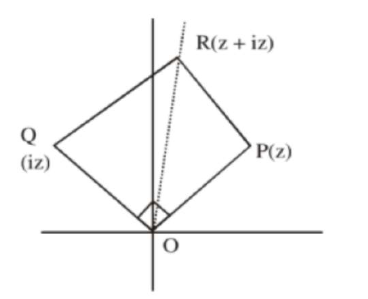# The area of the triangle with verticesQuestion:

The area of the triangle with vertices $\mathrm{A}(\mathrm{z}), \mathrm{B}(\mathrm{iz})$ and $\mathrm{C}(\mathrm{z}+\mathrm{iz})$ is :

1. (1) 1

2. (2) $\frac{1}{2}|z|^{2}$

3. (3) $\frac{1}{2}$

4. (4) $\frac{1}{2}|\mathrm{z}+\mathrm{iz}|^{2}$

Correct Option: , 3

Solution:$A=\frac{1}{2}|z||i z|$

$=\frac{|z|^{2}}{2}$